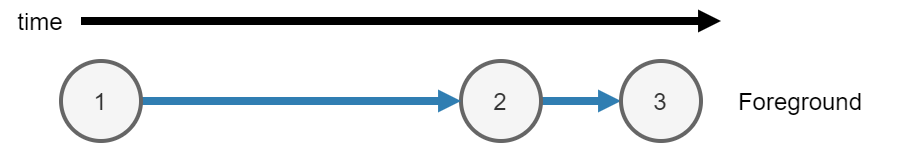Asynchronous Functions

MATLAB® either runs code synchronously or asynchronously. You can use the following functions to run code asynchronously:

Calculate the maximum of two random matrices. MATLAB runs each line consecutively.

tic
A = rand(10000);
B = ones(10000);
C = max(A,B);
toc
Elapsed time is 0.992156 seconds.

When you run the code, MATLAB runs three calculations.

1. Calculate A = rand(10000) in the foreground.

2. Calculate B = ones(10000) in the foreground.

3. After creating A and B, calculate C = max(A,B) in the foreground.Asynchronous Code

When you run a function asynchronously, MATLAB can run other code in the foreground at the same time.

Use parfeval or parfevalOnAll to run functions asynchronously. Use afterEach and afterAll to run functions asynchronously after a previous function completes.

• When you run a function asynchronously, MATLAB immediately returns a Future object. MATLAB schedules the function to run in the background or on a parallel pool.

• You can run other code while the function is running in the background.

• Use fetchOutputs to fetch results from the Future object.

Synchronous FunctionsAsynchronous Functions
MATLAB waits for the function to complete.MATLAB does not wait for the function to complete.
MATLAB runs the code immediately.MATLAB runs the code when a worker is available. For more information, see Background Workers.
Outputs from the function are available in the current workspace.To copy outputs to the current workspace, use fetchOutputs. When you use fetchOutputs, MATLAB waits for the function to complete.
You can use any function or object in a synchronous function.

You can use most functions and objects in an asynchronous function.

The current workspace is also available in a synchronous function.Most of the variables in the current workspace are also available in an asynchronous function.

Calculate the maximum of two random matrices: one created in the background, and one created in the foreground. Matrix A is created in the background, and matrix B is calculated in the foreground at the same time.

Note

Generally, you do not need to use wait. fetchOutputs will implicitly wait for MATLAB to finish running the function in the background before collecting results. The function wait is used here to explicitly show waiting for results before collecting them.

tic
fA = parfeval(backgroundPool,@rand,1,10000);
B = ones(10000);
wait(fA)
C = max(fetchOutputs(fA),B);
toc
Elapsed time is 0.534475 seconds.

When you run the code, MATLAB runs three calculations.

1. Calculate A = rand(10000) in the background.

1. Use parfeval to schedule the function rand to run in the background, with 1 output and a single input 10000. Return a future fA in the foreground.

2. Run the function rand in the background.

2. Calculate B = ones(10000) in the foreground.

3. After creating A and B, calculate C = max(A,B) in the foreground.

1. Use wait to explicitly wait for the future fA to finish running in the background.

2. Use fetchOutputs to get rand(10000) from the future fA.

3. Calculate the final result C from matrices fetchOutputs(fA) and B.Background Workers

When you use parfeval or parfevalOnAll to run a function asynchronously, MATLAB runs the function on a pool.

When you use backgroundPool to run code in the background, MATLAB uses the background pool to run that code.

The background pool has a fixed number of workers. MATLAB uses these workers to run functions. Each worker can only run one function at a time. Therefore when you run multiple functions in the background, you must wait for a worker to be available to run each function.

Use the NumWorkers property of a BackgroundPool to find out how many workers you have.

• If you do not have Parallel Computing Toolbox, the background pool has 1 worker.

• If you have Parallel Computing Toolbox, the background pool has multiple workers. The number of workers in the background pool is min(8,N) where N is the number of physical cores on your machine. For example if your machine has 4 cores, the background pool has 4 workers. If your machine has 16 cores, the background pool has 8 workers.